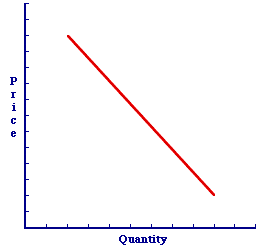Wednesday  October 28, 2020
 AmosWEB means Economics with a Touch of Whimsy!AGGREGATE PRODUCTION FUNCTION: A relation between the total production of real output for an economy and the amount of labor input. The aggregate production function is comparable to the standard production function used in the microeconomic analysis of firm behavior but is applied to the macroeconomic study of aggregate supply, resource markets, and employment. It is typically assumed to experience diminishing marginal returns, resulting in a decreasing marginal product of labor.CHANGE IN DEMAND:

A shift of the demand curve caused by a change in one of the demand determinants. A change in demand is caused by any factor affecting demand EXCEPT price. A related, but distinct, concept is a change in quantity demanded.
A change in demand is a change in the entire price-quantity relation that makes up the demand curve. It means that a different quantity demanded is paired with a given demand price or that a different demand price is paired with a given quantity demanded. The result of this repairing of prices and quantities is a repositioning, or a shift, of the demand curve.

The five demand determinants (buyers' income, buyers' preferences, other prices, buyers' expectations, and number of buyers) are responsible for causing a change in demand. In fact, the only thing that DOES NOT cause a change in demand is the demand price.

### Demand and Quantity Demanded

To set the stage for an understanding of this difference, take note of two related concepts:
• Demand: Demand is the range of quantities that buyers are willing and able to buy at a range of demand prices. It is ALL points that make up a demand curve.

• Quantity Demanded: Quantity demand is a specific quantity that buyers are willing and able to buy at a specific demand price. It is but ONE point on a demand curve.

### Making Changes

So what happens when the phrase "change in" is placed in front of each term?
• Change in Demand: A change in demand is a change in the ENTIRE demand relation. This means changing, moving, and shifting the entire demand curve. The entire set of prices and quantities is changing. In other words, this is a shift of the demand curve. A change in demand is caused by a change in the five demand determinants.

• Change in Quantity Demanded: A change in quantity demanded is a change from one price-quantity pair on an existing demand curve to a new price-quantity pair on the SAME demand curve. In other words, this is a movement along the demand curve. A change in quantity demanded is caused by a change in price.

### Changing Demand

A Change in DemandA change in demand is a shift of the demand curve. A change in quantity demanded is a movement along a given demand curve. These alternatives can be illustrated with the negatively-sloped demand curve presented in this exhibit. This demand curve captures the specific one-to-one, law of demand relation between demand price and quantity demanded. The five demand determinants are assumed to remain constant with the construction of this demand curve.
• A Change in Demand: A change in demand, which is triggered by a change in any of the five demand determinants, is a shift of the demand curve. Click the [A Determinant Change] button to demonstrate.

• A Change in Quantity Demanded: A change in quantity demanded, which is only triggered by a change in demand price, is a movement along the demand curve. Click the [A Price Change] button to demonstrate.

### An Important Difference

Why is this difference so important? The answer is as simple as cause and effect. The demand curve is used (together with supply) to explain and analyze market exchanges. The sequence of events follows a particular pattern.
• First, a demand (or supply) determinant changes.

• Second, this determinant change causes the demand curve (or supply curve) to shift.

• Third, the change in demand (or supply) causes either a shortage or a surplus imbalance in the market. The market is in a temporary state of disequilibrium.

• Fourth, the shortage and surplus imbalance causes the price of the good to change.

• Fifth, the change in price causes a change in quantity demanded (and supplied).

• Sixth, the change in quantity demanded (and supplied) eliminates the shortage or surplus and restores market equilibrium.

The key conclusion is that demand (and supply) determinants, which induce changes in demand (and supply), are the source of instability in the market. The change in price, which induces a change in quantity demanded (and supplied) is the means of eliminating the instability and restoring equilibrium.

 <= CHANGE IN AGGREGATE SUPPLY CHANGE IN PRIVATE INVENTORIES =>Recommended Citation:

CHANGE IN DEMAND, AmosWEB Encyclonomic WEB*pedia, http://www.AmosWEB.com, AmosWEB LLC, 2000-2020. [Accessed: October 28, 2020].

Check Out These Related Terms...

Or For A Little Background...

And For Further Study...
Search Again?BLUE PLACIDOLA[What's This?] Today, you are likely to spend a great deal of time looking for a downtown retail store seeking to buy either several magazines on home repairs or a remote controlled sports car with an air spoiler. Be on the lookout for cardboard boxes.Your Complete ScopeThe Dow Jones family of stock market price indexes began with a simple average of 11 stock prices in 1884."The less secure a man is, the more likely he is to have extreme prejudices. "-- Clint Eastwood, actor, directorPPTPersonal Property TaxA PEDestrian's Guide Xtra CreditTell us what you think about AmosWEB. Like what you see? Have suggestions for improvements? Let us know. Click the User Feedback link.| | | | | | | | | | |
| | | |

Thanks for visiting AmosWEB